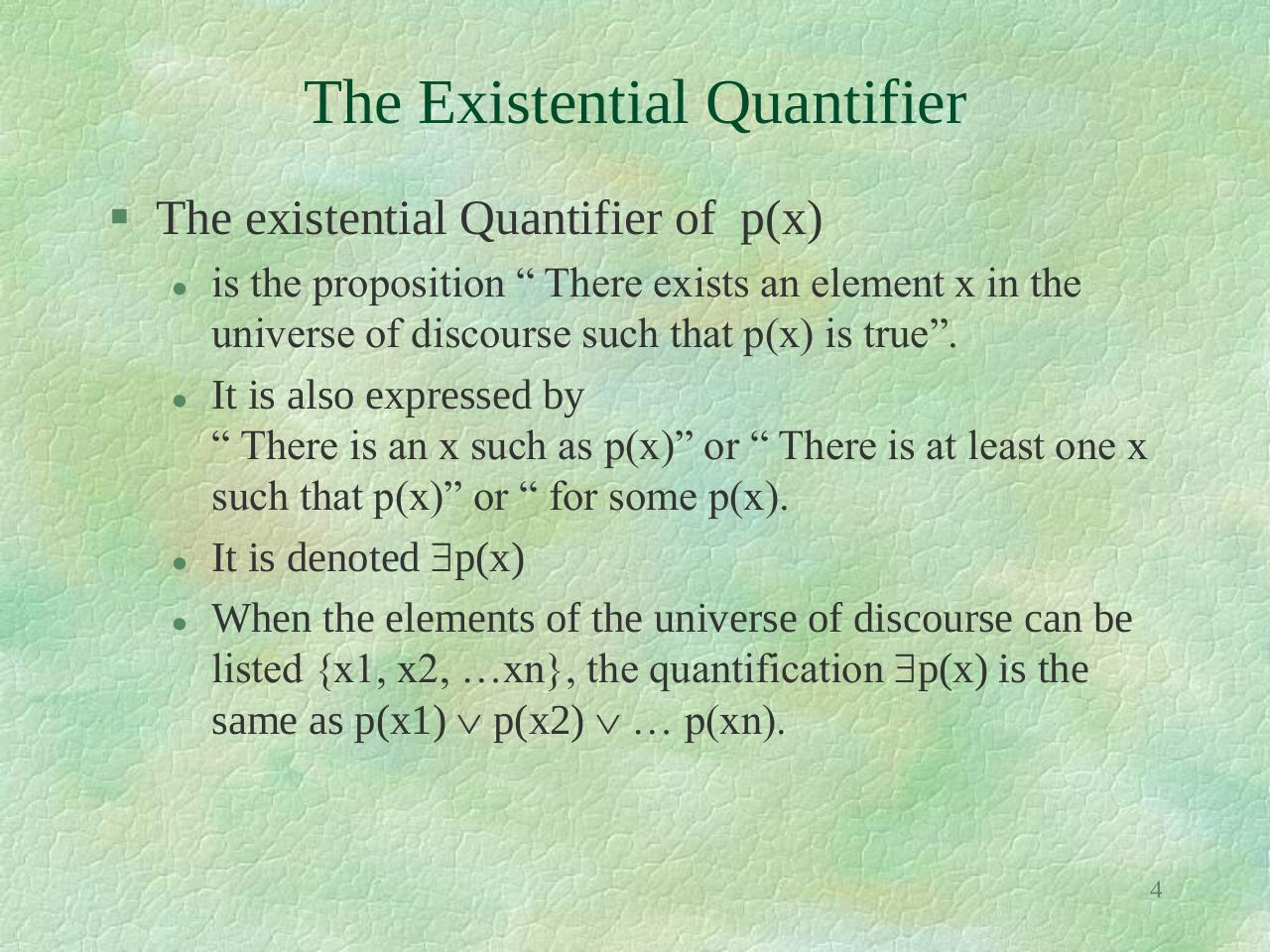Discreet lec 3.pdfPage 1 2 3 4 5 6 7 8 9 10

Text preview

The Existential Quantifier
 The existential Quantifier of p(x)

is the proposition “ There exists an element x in the
universe of discourse such that p(x) is true”.
It is also expressed by
“ There is an x such as p(x)” or “ There is at least one x
such that p(x)” or “ for some p(x).
It is denoted p(x)
When the elements of the universe of discourse can be
listed {x1, x2, …xn}, the quantification p(x) is the
same as p(x1)  p(x2)  … p(xn).

4